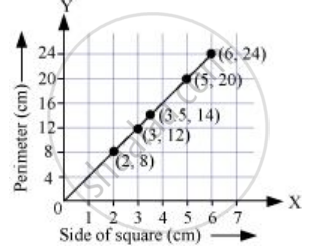Share

# Draw a Graph for the Following. Side of Square (In Cm) and Perimeter (In Cm) Is It a Linear Graph? - CBSE Class 8 - Mathematics

ConceptSome Applications

#### Question

Draw a graph for the following.

 Side of square (in cm) 2 3 3.5 5 5 Perimeter (in cm) 8 12 14 20 24

Is it a linear graph?

#### Solution

Choosing a suitable scale,

For x-axis, 1 unit = 1 cm and for y-axis, 1 unit = 4 cm

We can represent the side of a square on x-axis and the perimeter of that square on y-axis. A graph of the given data is drawn as follows.It is a linear graph.

Is there an error in this question or solution?

#### APPEARS IN

NCERT Solution for Mathematics Textbook for Class 8 (2018 to Current)
Chapter 15: Introduction to Graphs
Ex. 15.30 | Q: 2.1 | Page no. 248
Solution Draw a Graph for the Following. Side of Square (In Cm) and Perimeter (In Cm) Is It a Linear Graph? Concept: Some Applications.
S